Courses

# Test; Moment Of A Force On A Specified Axis

## 15 Questions MCQ Test Engineering Mechanics | Test; Moment Of A Force On A Specified Axis

Description
This mock test of Test; Moment Of A Force On A Specified Axis for Mechanical Engineering helps you for every Mechanical Engineering entrance exam. This contains 15 Multiple Choice Questions for Mechanical Engineering Test; Moment Of A Force On A Specified Axis (mcq) to study with solutions a complete question bank. The solved questions answers in this Test; Moment Of A Force On A Specified Axis quiz give you a good mix of easy questions and tough questions. Mechanical Engineering students definitely take this Test; Moment Of A Force On A Specified Axis exercise for a better result in the exam. You can find other Test; Moment Of A Force On A Specified Axis extra questions, long questions & short questions for Mechanical Engineering on EduRev as well by searching above.
QUESTION: 1

### Determine the moment’s magnitude produce by the force as shown in the diagram, which tends to rotate the rod ORQP along QP.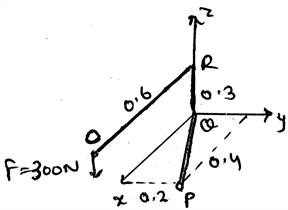Solution:

The use of the formula A.(rxF) gives the answer. In which A is 0.89i + 0.447j m. And the force is 300N, which is being applied at the end of the rod. Thus, after finding the equation of the axis and then replacing it in the equation shown above we get the answer. Actually, the main task is to know the axis equation in the vector form. Then get the magnitude of the moment.

QUESTION: 2

### The calculation of the moment about the axis and the moment about any point by a force applied on the body are different from each other.

Solution:

The calculation of the moment about the axis and the moment about any point by a force applied on the body are different from each other. It is because both of the calculations require different formulas. And both of the formulas have different inputs, which is obviously different.

QUESTION: 3

### In the calculation of the moment of the force about the axis, the cross product table, i.e. the 3X3 matrix which is made for doing the cross product having 3 rows, contains three elements. Which are they from top to bottom?

Solution:

The 3X3 matrix which is being made is having axis coordinates, point coordinates and the force coordinates. They are from top to bottom placed. The order cannot be changed. Or if changed then one needs to apply the negative sign appropriately. Negative because the directions gets reversed.

QUESTION: 4

Which of the following is correct? (For A representing the vector representation of the axis of rotation, r the radius vector and F the force vector)

Solution:

The correct form of the equation is given by A.(rxF). Where A represents the vector representation of the axis of rotation, r the radius vector and F the force vector. This is usually done for determining the moment of the force about the axis. That is if body is being rotated by the force about an axis.

QUESTION: 5

In the equation A.(rxF), the r vector is what?

Solution:

The r in the equation A.(rxF) is the radius vector. That is it is the vector which is having the start point at the axis and the end point at the point of action of the force on the body. This vector is being crossed by the force vector, which is then followed by the dot product with the axis vector.

QUESTION: 6

The axis vector in the calculation of the moment along the axis of rotation is the axis which is collinear with the force vector.

Solution:

The axis of the rotation cannot be collinear with the force vector. If it does so then the rotation of body is not possible. That is the moment of the force is zero. Which means no rotation being given by the force along the axis of rotation of the body.

QUESTION: 7

Which of the following is correct?

Solution:

As moment is a vector, we can represent it in the vector form easily. Whether it may be the moment of the force acting on the body about the axis, or about the point. We can also convert the same in the Cartesian form too. The only thing we need to do is the cross product of the radius vector and the force vector.

QUESTION: 8

What if the perpendicular distance from the axis is infinity?

Solution:

The long distance means a huge force which one needs to apply. Because distance increased will also increase the resistance inertia which will obviously increase the force required for the rotation. Though we know that the larger the distance the small is the force applied for rotation. But inertia must be taken into the considerations some times.

QUESTION: 9

In the equation A.(rxF) the r is heading from ______________ and ending at _____________

Solution:

It is the radius vector. The radius vector is always from the axis of rotation to the point of action of the force on the body. Which means that the radius vector is not on any point on the force vector. Rather it ending at the point on the force vector, where it is being in contact of the body.

QUESTION: 10

What if the moment of the force calculated about the axis is negative?

Solution:

It means that the direction of the motion is in the opposite sense as imagined. We can’t say about the direction of the force or the direction of the radius vector. But yes we can say about the direction of the rotation as it the thing which is going to be calculated. Rest all the parts are fixed. They can’t be altered.

QUESTION: 11

Determine the moment MQP in the vector form produce by the force as shown in the diagram, which tends to rotate the rod ORQP along QP.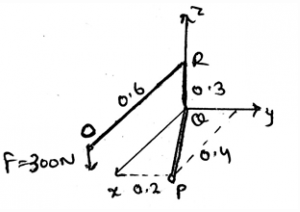Solution:

The use of the formula A.(rxF) gives the answer. In which A is 0.89i + 0.447j m. And the force is 300N, which is being applied at the end of the rod. Thus, after finding the equation of the axis and then replacing it in the equation shown above we get the answer. Actually, the main task is to know the axis equation in the vector form.

QUESTION: 12

Determine the moment of the force F along the segment QP of the pipe assembly shown in the figure.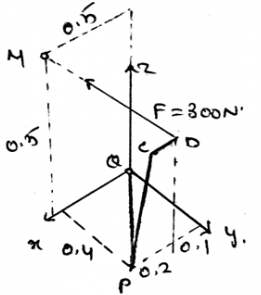Solution:

The use of the formula A.(rxF) gives the answer. In which A is 0.6i + 0.8j m and the r is 0.5i + 0.5k. And the force is 300N, which is being applied at the end of the rod. Thus, after finding the equation of the axis and then replacing it in the equation shown above we get the answer. Actually, the main task is to know the axis equation in the vector form. Then get the magnitude of the moment.

QUESTION: 13

Determine the magnitude of the moment of the force about the axis PQ.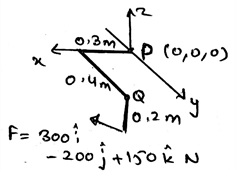Solution:

The use of the formula A.(rxF) gives the answer. In which A is 0.6i + 0.8j m and the r is -0.2k, and the force which is being applied at the end of the rod. Thus, after finding the equation of the axis and then replacing it in the equation shown above we get the answer. Actually, the main task is to know the axis equation in the vector form. Then get the magnitude of the moment.

QUESTION: 14

Determine the magnitude of the moment of the force about the y-axis.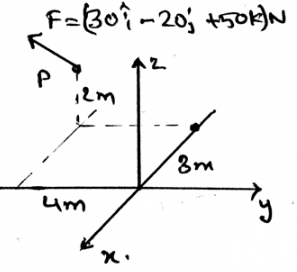Solution:

The use of the formula A.(rxF) gives the answer. In which A is 1j m and the r is -3i + 4j + 2k and the force which is being applied at the end of the rod. Thus, after finding the equation of the axis and then replacing it in the equation shown above we get the answer. Actually, the main task is to know the axis equation in the vector form. Then get the magnitude of the moment.

QUESTION: 15

The ___________ forces do not cause the rotation if the rotation is considered in about the axis of the body or the centroid axis of the body.

Solution:

The concurrent forces are the which are somewhere touching the axis of rotation. If any of the force is touching that axis, that force is not considered, or is insufficient to cause a rotation. If a force is concurrent then the perpendicular distance of the force from the line of axis is zero, thus no rotation. As we know rotation is caused by moment.